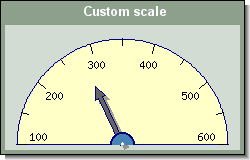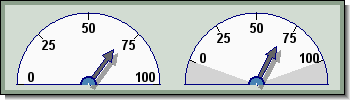## 20.3. Working with the odometer scale

In the previous section we ignored the actual scale in order to focus on the formatting options. Now we will do the (almost) opposite, only focus on how to manipulate the scale and the scale labels.

There are a number of properties that can be adjusted in the scale.

• The min and max values

• The fonts and for the scale

• The formatting of the scale values

• The start and end angles for the min and max values

• The tick interval

• The intervall of the scale labels

The scale is an instance of `class OdoScale` and is instantiated as an object that is accessed through the "\$scale" property of the plot

• `OdoPlot::scale`

### 20.3.1. Adjusting the scale, ticks and labels

By default the scale runs between 0 (inclusively) and 100 (inclusively). trying to set the indicator needle to a value outside this range will result in an error.

The min an max values o the scale are specified with the method

• `OdoScale::Set(\$aMin,\$aMax)`

The min/max values can be specified to any numeric value with the restriction that `\$aMin <= \$aMax`

To adjust which scale position should have tick marks and which tick marks should have a labels the following method is used.

• `OdoScle::SetTicks(\$aTickInterval, \$aLabelInterval)`

The first argument specifies the tick interval, i.e. the interval tick marks will be drawn in the odometer. The second argument specifies that every n:th tick should have a label.

The following line specifies that every 20 unit of the scale should have a tick mark and that every second tick mark should have a label

 ```1 ``` `\$odo->scale->SetTicks(20,2)`

### Tip

To make sure that both the min and max value of the scale gets a tick mark the tick multiplier should be an even divider to the scale range and the label multiplier should be a factor in the tick multiplier.

Figure 20.15 shows and example of how to change the scale, tick and label positions.

Figure 20.15.  `(odotutex14.php)`To adjust the appearance of the tick marks in respect to line weight, length and color the following three methods can be used:

• `OdoScale::SetTickLength(\$aLength)`

• `OdoScale::SetTickWeight(\$aWeight)`

• `OdoScale::SetTickColor('red')`

### Note

The tick length of a major tick mark (which have labels) are 1.5 times that of a minor tick mark. This factor can not be adjusted.

### 20.3.2. Adjusting scale label format

The following section will show hot to customize the appearance of scale and labels.

The scale labels can, as all other texts in the library have there font and color adjusted. The text property for the labels i accessed through the property

• `OdoScale::label`

Since this is a standard Text object we can adjust the font and color as the following example shows

 ```1 2 ``` ```\$odo->scale->label->SetFont(FF_FONT1,FS_BOLD); \$odo->scale->label->SetColor('navy');```

The way the labels are formatted are controlled by a format string with the standard "`printf()`" syntax, e.g "`%d`" (as default) displays an integer and "`%02f.1`" displays a label zero padded to two positions and with one decimal place.

The format string is applied by using the `OdoScale::SetLabelFormat()` method of the scale class as in

 ```1 ``` `\$odo->scale->SetLabelFormat('%d%%');`

The other aspect of the label formatting is exactly how the labels are positioned within the scale. By using the method

• `OdoScale::SetLabelPos(\$aPos)`

it is possible to specify the distance from the center to the label edge. By default this is 80% (i.e. specified as 0.8)

The following example shows the effect of applying some of these formatting options.

Example 20.4. Adjusting the scale format (`odotutex15.php`)

 ```1 2 3 4 5 6 7 8 9 10 11 12 13 14 15 16 17 18 19 20 21 22 23 24 25 26 27 28 29 30 31 32 33 34 35 36 37 38 39 40 41 42 43 44 45 46 ``` ```title->Set('Custom formatting'); \$graph->title->SetColor('white'); \$graph->title->SetFont(FF_ARIAL,FS_BOLD); // Add drop shadow for graph \$graph->SetShadow(); // Now we need to create an odometer to add to the graph. \$odo = new Odometer(); \$odo->SetColor("lightgray:1.9"); // Setup the scale \$odo->scale->Set(100,600); \$odo->scale->SetTicks(50,2); \$odo->scale->SetTickColor('brown'); \$odo->scale->SetTickLength(0.05); \$odo->scale->SetTickWeight(2); \$odo->scale->SetLabelPos(0.75); \$odo->scale->label->SetFont(FF_FONT1, FS_BOLD); \$odo->scale->label->SetColor('brown'); \$odo->scale->label->SetFont(FF_ARIAL,FS_NORMAL,10); // Setup a label with a degree mark \$odo->scale->SetLabelFormat('%dC'.SymChar::Get('degree')); // Set display value for the odometer \$odo->needle->Set(280); // Add drop shadow for needle \$odo->needle->SetShadow(); // Add the odometer to the graph \$graph->Add(\$odo); // ... and finally stroke and stream the image back to the browser \$graph->Stroke(); ?>```

Figure 20.16. Adjusting the scale format `(odotutex15.php)`### Note

As can be seen in Figure 20.16 we have used the utility `class SymChar` in order to get hold of the extended character that represents the degree mark. Remember that in order to get the extended character it is necessary to use a TTF scale.

### 20.3.3. Adjusting the start and stop angle for the scale

By default the scale labels start at 180 degree (9'a clock) and ends at 0 degree (3'a clock). To offer full flexibility this can be adjusted.

The method used for this is

• `OdoScale::SetAngle(\$aStart, \$aEnd)`

the angle is counted clockwise from the 6'a clock position. This means that the normal half odometer has a start angle of 90 degrees and an end angle of 270 degrees.

For full circle odometers the standard start angle is 40 degrees and the end angle is 320. This leaves a sector of 80 degrees free at the bottom of the odometer.

For example we could decide that the scale will start and stop 20 degrees above the horizontal line. The following example shows two odometer plots. The left uses the default start and end angle while the right odometer plot have adjusted start and end angles.

Figure 20.17. Adjusting start and end angles of scale `(odotutex16.php)`To further emphasize that the scale below the start and stop angles are "invalid" we could add a scale indicator in some gray:ish color that would signal that the area below 0 and 100 is not a valid scale area. We can do this by setting the range of the scale indicator to values outside the scale that would correspond to the horizontal line.

In Figure 20.17 we can accomplish this by adding the following two lines to the second odometer

 ```1 2 ``` ```\$odo2->AddIndication(-15,0,'lightgray'); \$odo2->AddIndication(100,115,'lightgray');```

The result of this is shown in Figure 20.18

Figure 20.18. Adding gray areas below the min and max scale values `(odotutex16.1.php)`